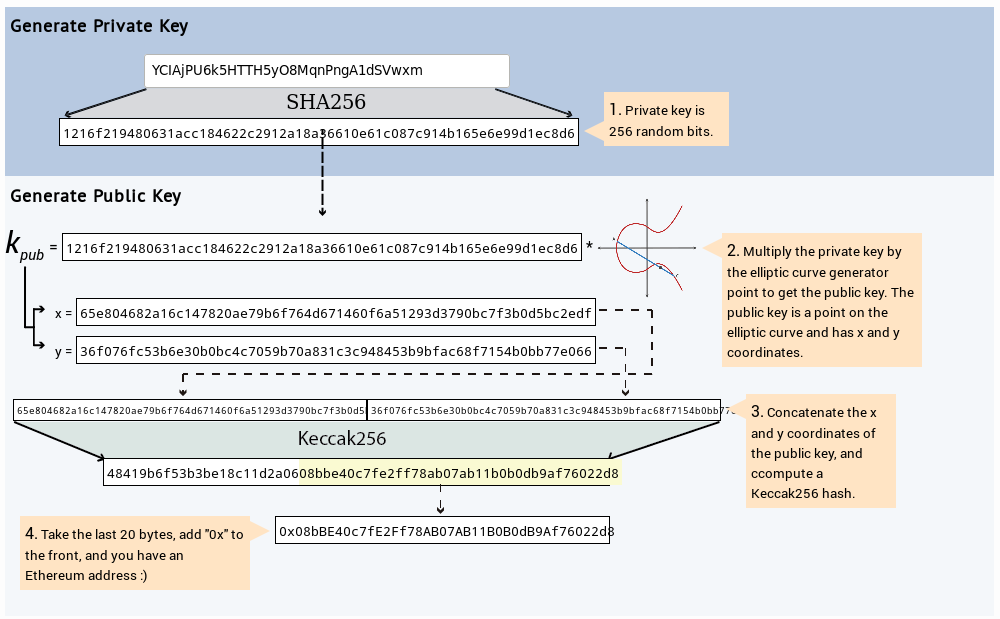Ethereum network enables developers to create smart contracts with an high level language and deploy them to the Ethereum network.

## Private and Public key

Private and public keys on ethereum blockchain, what are they? Private and public keys identify an Ethereum account (EOA). Private key is needed to sign transactions on the blockchain. Creating an ethereum account is creating a key pair: public key + private key. In the following discussion, we will see how a private key is generated and how the public one (and then the public address) is derived from the private key itself.

A private key is generated by random activity (machine generated randomness). Ethereum software uses the underlying OS’s random generator to produce 256 random bits (64 digits hexadecimal string)

In programming terms for getting a private key, we feed a larger string of random bits (from a machine-generated source of randomness) into a 256-bits hashing algorithm such as Keccak-256 or Sha-256. In this way we get a 256 bits hexadecimal string.

Private key generation is an offline process and does not require any interaction with Ethereum network. Never create or share your private key online.

A (random generated) private key is a 256 bits long and it is represented as an hexadecimal 64 digits string (64 digits x 4bits each). We can create for example a random number and then hash it by SHA-256, as we see in this below example

Create a machine generated 32 digits randomness, using Linux command line, and get:

massmux@massmux:~\$ cat /dev/urandom | tr -dc ‘a-zA-Z0-9’ | fold -w 32 | head -n 1

YCIAjPU6k5HTTH5yO8MqnPngA1dSVwxm

then feed the random string just generated to the sha-256 hashing function, in order to get the 256bits results (hex string 64 digits long)

massmux@massmux-golem:~\$ echo -n YCIAjPU6k5HTTH5yO8MqnPngA1dSVwxm | sha256sum

1216f219480631acc184622c2912a18a36610e61c087c914b165e6e99d1ec8d6

## The Public key

For calculating the public key, the elliptic curve aritmetic is necessary. An Ethereum public key is a point on the elliptic curve. This means that it is a set of x and y coordinates which satisfy the elliptic curve equation. The public key is composed by 2 numbers joined. They are produced from the private key. You cannot calculate, instead, the private key from public one. Getting in deeper on the elliptic curve is not in the scope of this article.The derivation procedure for the public key can be summarized as follows:

• create the private key, by generating a random 256 bits hex string by hashing (sha-256 or keccak-256) a source of randomness (as previously seen);
• multiply the private key by the elliptic curve generator point to get the public key; The public key is a point on the elliptic curve and has x and y coordinates;
• concatenate the x and y coordinates of the public key;